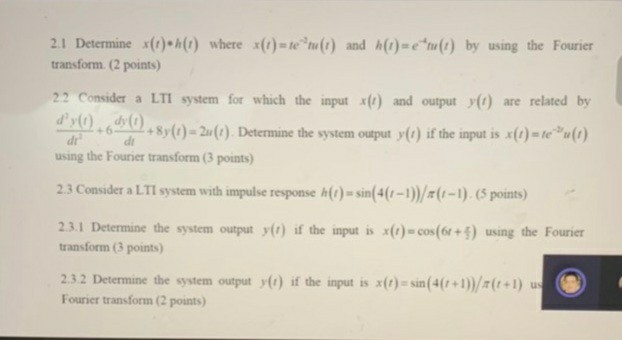# Question 2.1 Determine *(t)-h(1) where *(t) = te "tu(t) and h(t)=etu(t) by using the Fourier transform. (2 points) 22. Consider a LTI system for which the input x(!) and output y) are related by d'y().do(1) 487(0) 2u(). Determine the system output y(t) if the input is *(0) = reu(1). dr using the Fourier transform (3 points) 2.3 Consider a LTI system with impulse response h(t) = sin(4(1-1))/=(1-1). (5 points) 2.3.1 Determine the system output y() if the input is x(1) - cos(65+) using the Fourier transform (3 points) 2.3.2 Determine the system output y() if the input is x(t) = sin(4(1+1))/(t+1) us Fourier transform (2 points)BMN6TO The Asker · Electrical EngineeringTranscribed Image Text: 2.1 Determine *(t)-h(1) where *(t) = te "tu(t) and h(t)=etu(t) by using the Fourier transform. (2 points) 22. Consider a LTI system for which the input x(!) and output y) are related by d'y().do(1) 487(0) 2u(). Determine the system output y(t) if the input is *(0) = reu(1). dr using the Fourier transform (3 points) 2.3 Consider a LTI system with impulse response h(t) = sin(4(1-1))/=(1-1). (5 points) 2.3.1 Determine the system output y() if the input is x(1) - cos(65+) using the Fourier transform (3 points) 2.3.2 Determine the system output y() if the input is x(t) = sin(4(1+1))/(t+1) us Fourier transform (2 points)
More
Transcribed Image Text: 2.1 Determine *(t)-h(1) where *(t) = te "tu(t) and h(t)=etu(t) by using the Fourier transform. (2 points) 22. Consider a LTI system for which the input x(!) and output y) are related by d'y().do(1) 487(0) 2u(). Determine the system output y(t) if the input is *(0) = reu(1). dr using the Fourier transform (3 points) 2.3 Consider a LTI system with impulse response h(t) = sin(4(1-1))/=(1-1). (5 points) 2.3.1 Determine the system output y() if the input is x(1) - cos(65+) using the Fourier transform (3 points) 2.3.2 Determine the system output y() if the input is x(t) = sin(4(1+1))/(t+1) us Fourier transform (2 points)